Next: F Second Order Co Up: Dissertation Christoph Wasshuber Previous: D Integration of Fermi

# E Fermi's Golden Rule

The time dependent Schrödinger Equation can be written as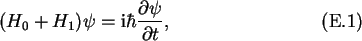where H1 is a small perturbation to the Hamiltonian H0. The solution of the unperturbed case is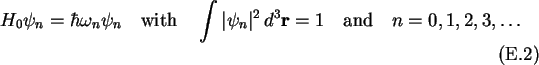The eigenvalues are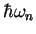, and the orthonormal eigenfunctions are. Under the assumption that the perturbation H1 is small, the wave function for the perturbed case can be expanded in a series of the orthonormal wave functions of the unperturbed case .Inserting (E.3) into the time dependent Schrödinger equation (E.1), and using (E.2) gives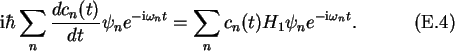If this is multiplied by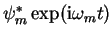, and integrated over the volume one obtains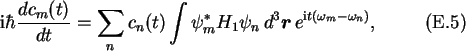since orthonormality makes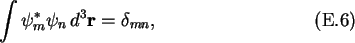which vanishes for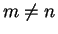and equals unity for m=n. With the definitions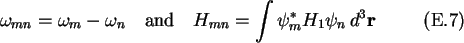one can write (E.5) as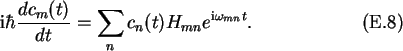In general, when the perturbation is turned on at t=0, it may be assumed that the system is in state m at t=0, and cm(0)=1, while cn(0)=0for all other states. Furthermore, it is assumed that the scattering out of the initial state is small, so that cm(t)=1 for all time. This assumption neglects the  conservation of particles. Considering these assumptions, one can solve (E.8).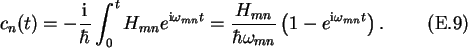The probability of occupying state n is then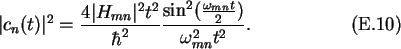For times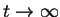, which are long enough such that the scattering process has been completed, the functionhas the property of a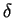-function (see Fig. E.1).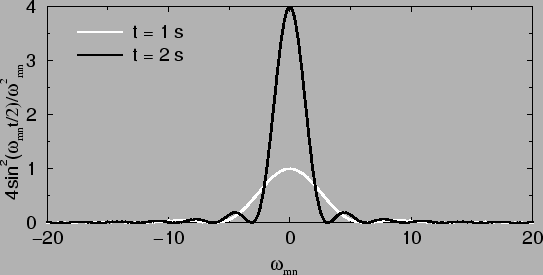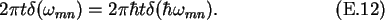The transition probability per unit time, the   transmission rate, is then given by the so called Fermi Golden Rule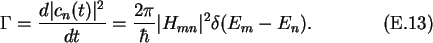This means that a scattering process, in our case a tunnel event, only takes place if the energy of the particle is conserved, Em = En.Next: F Second Order Co Up: Dissertation Christoph Wasshuber Previous: D Integration of Fermi

Christoph Wasshuber